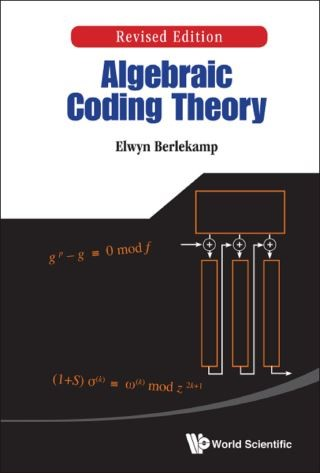## Algebraic Coding Theory (Revised Edition)• Description
• Author
• Info
• Reviews

### Description

This is the revised edition of Berlekamp's famous book, "Algebraic Coding Theory", originally published in 1968, wherein he introduced several algorithms which have subsequently dominated engineering practice in this field. One of these is an algorithm for decoding Reed-Solomon and Bose–Chaudhuri–Hocquenghem codes that subsequently became known as the Berlekamp–Massey Algorithm. Another is the Berlekamp algorithm for factoring polynomials over finite fields, whose later extensions and embellishments became widely used in symbolic manipulation systems. Other novel algorithms improved the basic methods for doing various arithmetic operations in finite fields of characteristic two. Other major research contributions in this book included a new class of Lee metric codes, and precise asymptotic results on the number of information symbols in long binary BCH codes. Selected chapters of the book became a standard graduate textbook. Both practicing engineers and scholars will find this book to be of great value.Contents:

• Basic Binary Codes
• Arithmetic Operations Modulo An Irreducible Binary Polynomial
• The Number of Irreducible q-ary Polynomials of Given Degree
• The Structure of Finite Fields
• Cyclic Binary Codes
• The Factorization of Polynomials Over Finite Fields
• Binary BCH Codes for Correcting Multiple Errors
• Nonbinary Coding
• Negacyclic Codes for the Lee Metric
• Gorenstein-Zierler Generalized Nonbinary BCH Codes for the Hamming Metric
• Linearized Polynomials and Affine Polynomials
• The Enumeration of Information Symbols in BCH Codes
• The Information Rate of the Optimum Codes
• Codes Derived by Modifying or Combining Other Codes
• Other Important Coding and Decoding Methods
• Weight Enumerators
• Appendices and References
Researchers in coding theory and cryptography, algebra and number theory, and software engineering. Key Features:
• A classic monograph and reference book on Coding Theory and Cryptography written by a revered scholar long known for his work in coding theory
• Contains several theorems created by the author that have been respected for 40 years